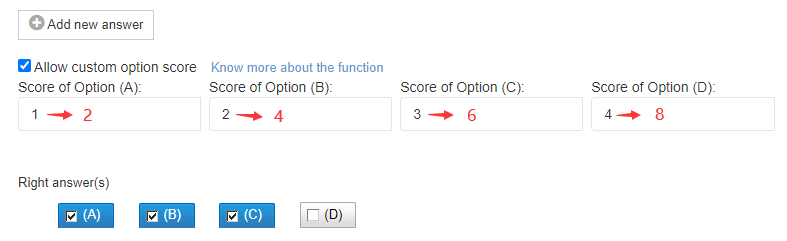# Exams Online Testing Platform - Online Exam Platform

## Instructions of the Settings for Exam Scores

When adding test questions to the exams, the scores of the questions default to the scores set during import. But users can modify the score of each question.

The instructions for score modification are as follows:

1. The questions imported into the system can be used in multiple exams. If the score of a question is modified in a certain exam, it is only modified in this exam, and will not affect the score of other exams and the score of the question in the question bank.

2. If there is a need to revise the sore of a question in a certain exam, the score will follow these rules:

(1) After revising the question scores,  the revised scores will be used instead of the score in the question bank online.

For example, if a question is originally 2 points, and the score of the question is changed to 10 when forming an exam, The candidates will get 10 points if they answer this question correctly.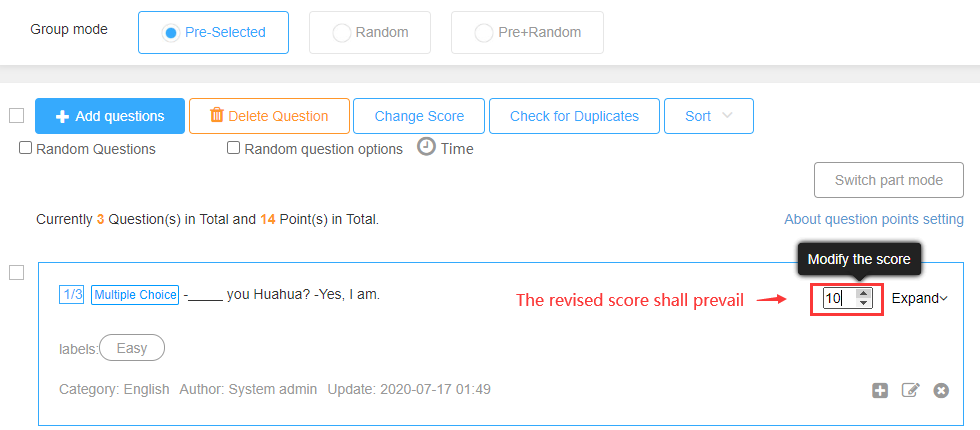Settings for Exam Scores

(2) If a question is set to be scored by blanks, after revising the scores, the system will allocate the score of each blank proportionally.

For example, there are three blanks in a question, and the original scores for each space are 2, 2, and 1 (The total score is 5 points.)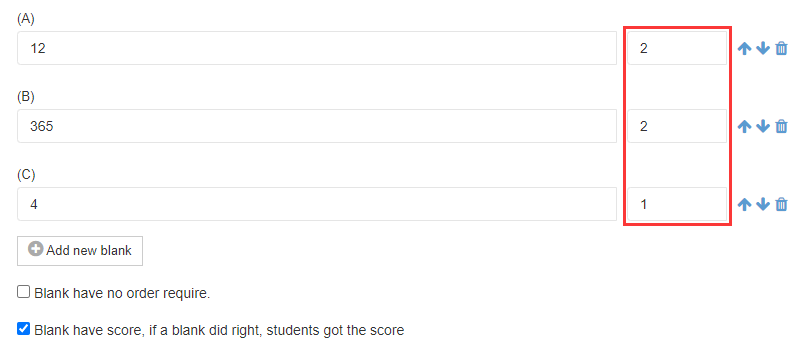question bank online

When the total score is changed from 5 to 10 when forming an exam, each blank will be calculated proportionally and the score will turn into 4, 4 and 2.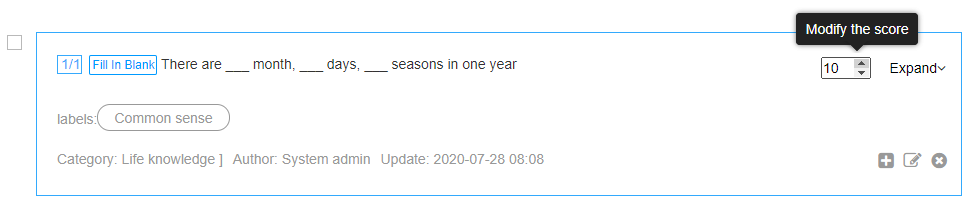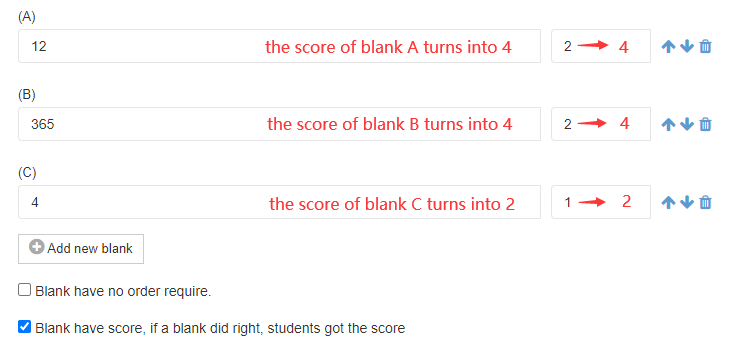(3)When the multiple choice questions allow custom option score, the system will assign the score of each option proportionally after revising the scores.

For example, the score of a multiple choice question is customized to 1, 2, 3 and 4 (with a total score of 10). If the total score is changed to 20, the score of the four options will turn into 2, 4, 6 and 8.